﻿ 考虑直流电网关键设备的限流电抗优化配置方法 Optimal Configuration Method of Reactor Considering the Key Equipment in DC Grid

Smart Grid
Vol. 11  No. 01 ( 2021 ), Article ID: 40586 , 11 pages
10.12677/SG.2021.111006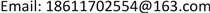Optimal Configuration Method of Reactor Considering the Key Equipment in DC Grid

Xinfu Sun, Jun Yan, Chengyong Zhao

State Key Laboratory of Alternate Electrical Power System with Renewable Energy Sources (North China Electric Power University), BeijingReceived: Jan. 21st, 2021; accepted: Feb. 13th, 2021; published: Feb. 24th, 2021ABSTRACT

The rapid clearing of DC faults is the key to ensuring the continuous operation of the grid. Equipments such as Current Limited Reactor (CLR), Fault-Current-Limiter (FCL) and hybrid DCCB can weaken the development speed of fault current. At the same time, they also have influence on the development characteristics of fault current. Further research is needed to make the configuration of CLR and FCL more reasonable. Firstly, the current calculation method of DC grid is given considering the energy absorption of FCL and the action sequence of DCCB. Secondly, taking the breaking current of DCCB and the energy absorbed by current limiting equipment as optimization targets, the optimization method of the DC reactance is obtained. Finally, PSCAD/EMTDC simulation verified that the proposed optimization method reduces the fault breaking current by 15% compared with the previous optimization method.

Keywords:DC Power Grid, Energy Absorption, Fault Current Limiter, Action Sequence, Optimized Configuration1. 引言

(1) 限制故障后能量源馈入能量 。

(2) 提高故障开断速度 。

(3) 抑制故障电流上升率  。

2. 考虑并联MOA的限流电抗器吸能的故障等效建模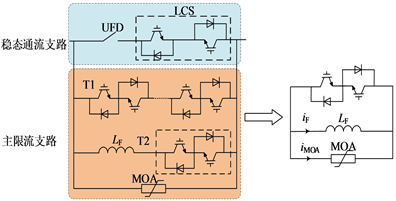Figure 1. Topology of fault current limiter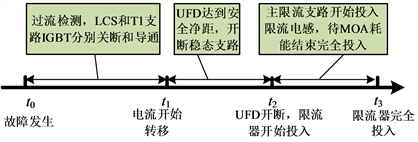Figure 2. Action sequence and principle of fault current limiter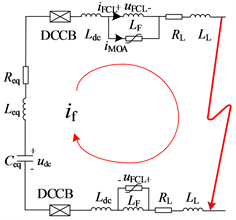Figure 3. Equivalent circuit after inputting current-limiting reactor LF

${i}_{\text{f}}={i}_{\text{MOA}}+{i}_{\text{F}}$ (1)

${u}_{\text{MOA}}\left({i}_{\text{MOA}}\right)={L}_{\text{F}}\frac{\text{d}\left({i}_{\text{f}}-{i}_{\text{MOA}}\right)}{\text{d}t}$ (2)

$\left\{\begin{array}{l}A\cdot u=R\cdot i+L\cdot \frac{\text{d}i}{\text{d}t}+{\left[{u}_{MOA1},{u}_{\mathrm{MOA}2},0,0,0\right]}^{\text{T}}\\ \frac{\text{d}u}{\text{d}t}=C\cdot i\text{}\\ \frac{\text{d}{i}_{\text{F}}}{\text{d}t}=\mathrm{diag}\left(\frac{1}{{L}_{F1}},\frac{1}{{L}_{F2}}\right)\cdot {\left[{u}_{MOA1},{u}_{\mathrm{MOA}2}\right]}^{\text{T}}\end{array}$ (3)

$C=-diag\left[\begin{array}{cccc}1/{C}_{\text{C1}}& 1/{C}_{\text{C}2}& 1/{C}_{\text{C3}}& 1/{C}_{\text{C4}}\end{array}\right]\cdot {A}^{\text{T}}$ (4)

$\left({L}_{\text{eq}}+{L}_{\text{dc}}+{L}_{\text{L}}\right)\cdot {i}_{\text{f}}\left({t}_{1-}\right)=\left({L}_{\text{eq}}+{L}_{\text{dc}}+{L}_{\text{L}}+{L}_{\text{F}}\right)\cdot {i}_{\text{f}}\left({t}_{1+}\right)$ (5)

3. 考虑混合式断路器动作时序的故障等效建模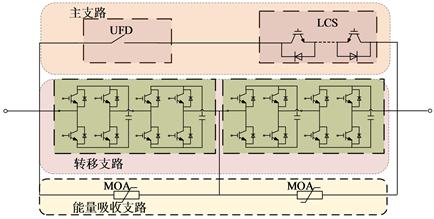Figure 4. Topology of the cascade full bridge Direct Current Circuit Breaker (DCCB)

t1时刻，主支路的机械开关达到安全开距，全桥子模块的下桥臂IGBT断开，故障电流开始向电容充电。此时断路器等效电路为多个子模块电容串联而成的等效小电容。经过约数十ms，在t2时刻电容电压充到MOA的钳位电压，故障电流转移到能量吸收支路(Energy Absorption Branch, EAB)。能量吸收过程应当位于金属氧化物V-I特性曲线的线性部分，意味着在这个阶段能量吸收支路等效为一个串联的直流电压源。因此t0-t1电路等效为小电阻，用标识O表示，t1-t2电路等效为电容，用标识C表示，t2-t3电路等效为电压源，用标识S表示，t3时刻以后，直流断路器开断，电路为开路，用标识B表示，动作时序如图5所示。

(1) CB1和CB2不动作状态(1O2O)

t1时刻之前，断路器等效为小电阻，可忽略，故障电流计算方程仍如文献  所示。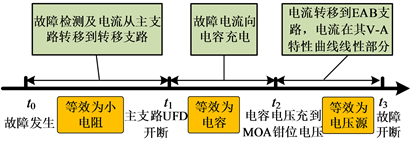Figure 5. Action sequence diagram of the cascade full bridge DCCB

$\left\{\begin{array}{l}A\cdot u=R\cdot i+L\cdot \frac{\text{d}i}{\text{d}t}\\ \frac{\text{d}u}{\text{d}t}=C\cdot i\end{array}$ (6)

(2) CB1和CB2等效为电容状态(1C2C)

$C=-diag\left[\begin{array}{llllll}1/{C}_{\text{C1}}\hfill & 1/{C}_{\text{CB1}}\hfill & 1/{C}_{\text{CB}2}\hfill & 1/{C}_{\text{C}2}\hfill & 1/{C}_{\text{C3}}\hfill & 1/{C}_{\text{C4}}\hfill \end{array}\right]\cdot {A}^{\text{T}}$ (7)

(3) CB1等效为直压源，CB2等效为电容阶段(1S2C)

$C=-diag\left[\begin{array}{lllll}1/{C}_{\text{C1}}\hfill & 1/{C}_{\text{CB}2}\hfill & 1/{C}_{\text{C}2}\hfill & 1/{C}_{\text{C3}}\hfill & 1/{C}_{\text{C4}}\hfill \end{array}\right]\cdot {A}^{\text{T}}$ (8)

$A\cdot u=R\cdot i+L\cdot \frac{\text{d}i}{\text{d}t}+{\left[\begin{array}{lllll}{u}_{\text{MOA1}}\hfill & 0\hfill & 0\hfill & 0\hfill & 0\hfill \end{array}\right]}^{\text{T}}$ (9)

(4) CB1和CB2均为直流电压源等效电路(1S2S)

$A\cdot u=R\cdot i+L\cdot \frac{\text{d}i}{\text{d}t}+{\left[\begin{array}{lllll}{u}_{\text{MOA1}}\hfill & {u}_{\text{MOA2}}\hfill & 0\hfill & 0\hfill & 0\hfill \end{array}\right]}^{\text{T}}$ (10)

(5) CB1断开CB2直流源状态(1B1S)

4. 考虑MOA的吸能过程和直流断路器动作时序的限流电抗优化

4.1. 优化目标函数

MOA中的能量可由式(11)计算得到：

${Q}_{\text{MOA}}={\int }_{0}^{{t}_{b}}{u}_{\text{MOA}}{i}_{\text{MOA}}\text{d}t$ (11)

$\begin{array}{l}\mathrm{min}F=\mathrm{min}\left(\underset{i=1}{\overset{n=8}{\sum }}{I}_{\text{b}},\underset{i=1}{\overset{n=8}{\sum }}{Q}_{\text{MOAS}i}\right)\\ \text{s}\text{.t}\text{.}\left\{\begin{array}{l}{I}_{\text{b}}\le 15\text{\hspace{0.17em}}\text{kA}\\ {I}_{\text{dcpk}}\le 18\text{\hspace{0.17em}}\text{kA}\\ {I}_{\text{b}}<{I}_{\text{dc}}\left(t=3.5\text{\hspace{0.17em}}\text{ms}\right)\\ {L}_{i=1~8}\ge 0.015\text{\hspace{0.17em}}\text{H}\end{array}\end{array}$ (12)

4.2. 多目标优化

$\begin{array}{l}\left\{\begin{array}{l}F={k}_{1}{f}_{1}+{k}_{2}{f}_{2}\\ {k}_{1}+{k}_{2}=1\end{array}\\ \mathrm{min}F\end{array}$ (13)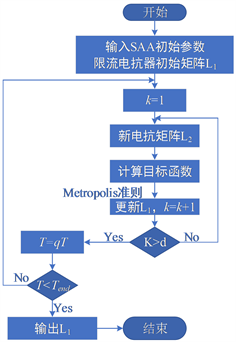Figure 6. SAA optimization process of current limiting reactor

$\begin{array}{l}\mathrm{min}{F}^{\prime }=\mathrm{min}F+M{\left(\mathrm{min}\left[15-{I}_{\text{b}},0\right]\right)}^{2}+\cdots \\ \text{ }\text{ }\text{ }\text{ }\text{ }\text{ }\text{ }\text{ }\text{ }\text{ }\text{ }\text{ }\text{ }\text{ }\text{ }\text{ }\text{ }\text{ }\text{ }\text{ }\text{ }\text{ }\text{ }\text{ }\text{ }\text{ }\text{ }\text{ }\text{ }\text{ }\text{ }\text{ }\text{ }\text{ }\text{ }\text{ }+M{\left(\mathrm{min}\left[18-{I}_{\text{dcpk}},0\right]\right)}^{2}+\cdots \\ \text{ }\text{ }\text{ }\text{ }\text{ }\text{ }\text{ }\text{ }\text{ }\text{ }\text{ }\text{ }\text{ }\text{ }\text{ }\text{ }\text{ }\text{ }\text{ }\text{ }\text{ }\text{ }\text{ }\text{ }\text{ }\text{ }\text{ }\text{ }\text{ }\text{ }\text{ }\text{ }\text{ }\text{ }\text{ }\text{ }+M{\left(\mathrm{min}\left[{I}_{\text{dcpk}}-{I}_{\text{b}},0\right]\right)}^{2}+\cdots \end{array}$ (14)

5. 仿真验证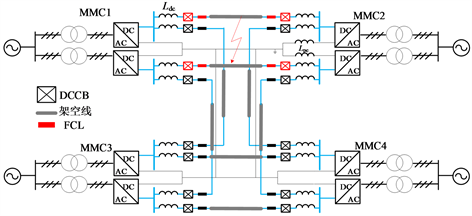Figure 7. Four-terminal DC grid topology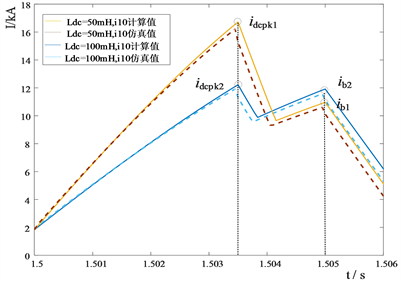(a) MMC1出口故障电流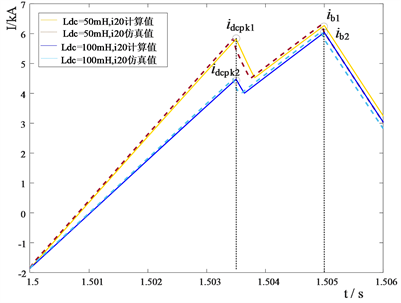(b) MMC2出口故障电流

Figure 8. DC fault current under different DC reactance combinationsTable 2. Energy Absorption by FCL and DCCB under Different CLRsTable 3. Optimization results of DC reactors under different weights

6. 结论

(1) 对故障限流器中的吸能元件能量吸收过程和故障两侧断路器动作时序进行建模，给出了考虑多种限流设备动作的直流电网故障电流计算方法。

(2) 利用模拟退火算法给出了直流电网各条线路上的限流电抗器优化配置的方法，保证了在经济成本一定的情况下，限流效果达到最优。为直流侧限流电抗器参数的优化配置提供了理论依据。

Optimal Configuration Method of Reactor Considering the Key Equipment in DC Grid[J]. 智能电网, 2021, 11(01): 47-57. https://doi.org/10.12677/SG.2021.111006

1. 1. 杨艳晨, 郭剑波, 王姗姗, 王铁柱, 李英彪, 赵兵, 吴广禄. 柔性直流电网直流过电压分析及控制策略研究[J]. 电网技术, 2019, 43(5): 1586-1592. http://dx.chinadoi.cn/10.13335/j.1000-3673.pst.2018.0588

2. 2. 孔明, 邱宇峰, 贺之渊, 何维国, 刘隽. 模块化多电平式柔性直流输电换流器的预充电控制策略[J]. 电网技术, 2011, 35(11): 67-73.

3. 3. 吴俊宏, 艾芊. 多端柔性直流输电系统在风电场中的应用[J]. 电网技术, 2009, 33(4): 22-27.

4. 4. 姚良忠, 吴婧, 王志冰, 李琰, 鲁宗相. 未来高压直流电网发展形态分析[J]. 中国电机工程学报, 2014(34): 6007-6020.

5. 5. 刘泽洪, 郭贤珊. 含新能源接入的双极柔性直流电网运行特性研究与工程实践[J]. 电网技术, 2020, 44(9): 3595-3603. http://dx.chinadoi.cn/10.13335/j.1000-3673.pst.2020.0254a

6. 6. 赵成勇, 宋冰倩, 许建中. 柔性直流电网故障电流主动控制典型方案综述[J]. 电力系统自动化, 2020, 44(5): 3-13. http://dx.chinadoi.cn/10.7500/AEPS20190626003

7. 7. Leterme, W., Beerten, J. and Van Hertem, D. (2016) Equivalent Circuit for Half-Bridge MMC dc Fault Current Contribution. 2016 IEEE International Energy Conference (ENERGYCON), Leuven, 4-8 April 2016, 1-6.
https://doi.org/10.1109/ENERGYCON.2016.7513914

8. 8. Callavik, M., Blomberg, A., Häfner, J. and Jacobson, B. (2012) The Hybrid HVDC Breaker An Innovation Breakthrough Enabling Reliable HVDC Grids. ABB Grid System, Technical Paper, 361, 143-152.

9. 9. Lin, W. and Jovcic, D. (2014) LCL and L-VSC Converters with DC Fault Current-Limiting Property and Minimal Power Losses. IEEE Transactions on Power Delivery, 29, 2359-2368.
https://doi.org/10.1109/TPWRD.2014.2314481

10. 10. Deng, F. and Chen, Z. (2013) Design of Protective Inductors for HVDC Transmission Line within DC Grid Offshore Wind Farms. IEEE Transactions on Power Delivery, 28, 75-83.
https://doi.org/10.1109/TPWRD.2012.2224384

11. 11. 杨汾艳, 徐政. 直流输电系统平波电抗器电感参数的选择研究[J]. 高压电器, 2009, 45(3): 8-10+14.

12. 12. 孙帮新. 特高压直流系统中平波电抗器配置方案[J]. 电工技术, 2009(11): 6-7+40.

13. 13. Kontos, E., Rodrigues, S., Pinto, R.T. and Bauer, P. (2014) Optimization of Limiting Reactors Design for DC Fault Protection of Multi-Terminal HVDC Networks. 2014 IEEE Energy Conversion Congress & Exposition, Pittsburgh, 14-18 September 2014, 5347-5354.
https://doi.org/10.1109/ECCE.2014.6954134

14. 14. 李岩, 龚雁峰. 多端直流电网限流电抗器的优化设计方案[J]. 电力系统自动化, 2018, 42(23): 120-128. http://dx.chinadoi.cn/10.7500/AEPS20180308009

15. 15. 王守相, 禚程程, 刘琪, 杨景刚, 陈庆. 基于VSC的直流配电网限流电抗器位置和参数优化配置方法[J]. 电力自动化设备, 2019, 39(12): 89-95. http://dx.chinadoi.cn/10.16081/j.epae.201911030

16. 16. 朱思丞, 赵成勇, 李承昱, 许建中. 考虑故障限流器动作的直流电网限流电抗器优化配置[J]. 电力系统自动化, 2018, 42(15): 142-149+281-283. http://dx.chinadoi.cn/10.7500/AEPS20180308007

17. 17. 李国庆, 边竞, 王鹤, 孙云鹤, 王振浩. 考虑直流电抗器的电容型限流器特性及优化配置[J]. 电力系统自动化, 2020, 44(5):23-29+234-241. http://dx.chinadoi.cn/10.7500/AEPS20190607003

18. 18. Lee, H.Y., Mansoor, A., Park, K.H. and Lee, B.-W. (2018) Feasible Application Study of Several Types of Superconducting Fault Current Limiters in HVDC Grids. IEEE Transactions on Applied Superconductivity, 28, Article No. 5601205.
https://doi.org/10.1109/TASC.2018.2799745

19. 19. Font, A., Ilhan, S. and Ozdemir, A. (2016) Line Surge Arrester Application for a 380 kV Power Transmission Line. 2016 IEEE International Conference on High Voltage Engineering & Application, Chengdu, 19-22 September 2016, 1-4.
https://doi.org/10.1109/ICHVE.2016.7800722

20. 20. Li, C.Y., Zhao, C.Y., Xu, J.Z., Ji, Y.K., Zhang, F. and An, T. (2017) A Pole-to-Pole Short-Circuit Fault Current Calculation Method for DC Grids. IEEE Transactions on Power Systems, 32, 4943-4953.
https://doi.org/10.1109/TPWRS.2017.2682110